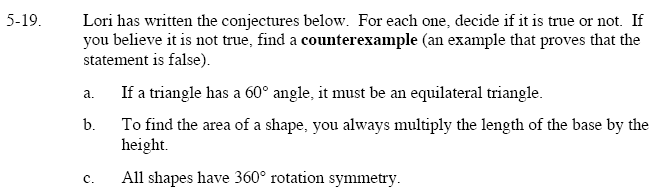### Home > GC > Chapter 5 > Lesson 5.1.2 > Problem5-19

5-19.
1. Lori has written the conjectures below. For each one, decide if it is true or not. If you believe it is not true, find a counterexample (an example that proves that the statement is false). Homework Help ✎

1. If a triangle has a 60° angle, it must be an equilateral triangle.

2. To find the area of a shape, you always multiply the length of the base by the height.

3. All shapes have 360° rotation symmetry.Can a scalene triangle have a 60° angle?

How do you find the area of a trapezoid?

True